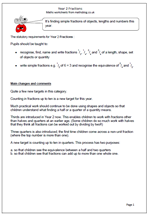# New Maths Curriculum: Year 2 FractionsFractions are seen as a completely separate area now, rather than being within Number or Division and Fractions.

The statutory requirements for Year 2 Fractions :

Pupils should be taught to:

•    recognise, find, name and write fractions 1/3, 1/4, 2/4 and 3/4 of a length, shape, set of objects or quantity

•    write simple fractions e.g. 1/2 of 6 = 3 and recognise the equivalence of 2/4 and 1/2.

Quite a few new targets in this category.
Counting in fractions up to ten is a new target for this year.
Much practical work should continue to be done using shapes and objects so that children understand what finding a half or a quarter of a quantity means.

Thirds are introduced in Year 2 now. This enables children to work with fractions other than halves and quarters at an earlier age. (Some children do so much work with halves that they think all fractions can be worked out by dividing by two!!)

Three quarters is also introduced; the first time children come across a non-unit fraction (where the top number is more than one).

A new target is counting up to ten in quarters. This process has two purposes:

a. so that children see the equivalence between a half and two quarters
b. so that children see that fractions can add up to more than one whole one.

New Maths Curriculum: Fractions